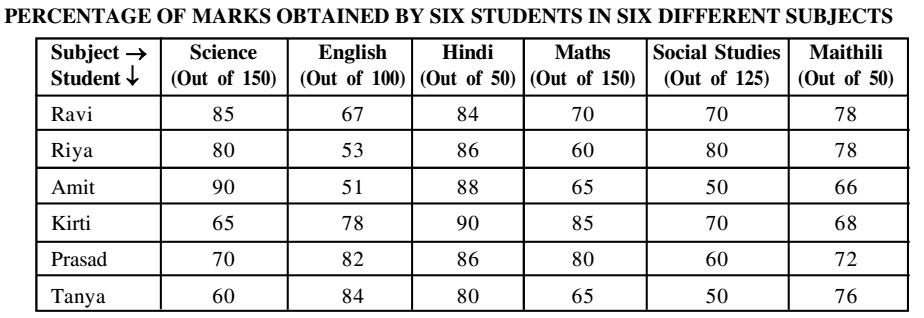## Table chart

#### Data Interpretation

Direction: Study the table carefully to answer the questions that follow:1. What are the average marks obtained by all students together in Hindi?

1. As per given table graph,
The total marks obtained by all students in Hindi = Marks obtained by ravi in Hindi + Marks obtained by riya in Hindi + Marks obtained by amit in Hindi +Marks obtained by kirti in Hindi + Marks obtained by prasad in Hindi + Marks obtained by tanya in Hindi

##### Correct Option: B

As per given table graph,
The total marks obtained by all students in Hindi = Marks obtained by ravi in Hindi + Marks obtained by riya in Hindi + Marks obtained by amit in Hindi +Marks obtained by kirti in Hindi + Marks obtained by prasad in Hindi + Marks obtained by tanya in Hindi
The total marks obtained by all students in Hindi = 50 x (84 + 86 + 88 + 90 + 86 + 80)/100 = 50 x 514/100 = 257
The average marks obtained by all students in Hindi = 257/6 = 42.83

1. If to pass in the examination the minimum marks rewired in Maths are 95 and in Social studies are 85, how many students will pass ?

1. Pass percentage in Maths = Minimum passing marks in Maths x 100/total maths marks for math
Pass percentage in Social studies = Minimum passing marks in Social studies x 100/total maths marks for Social studies

##### Correct Option: E

Pass percentage in Maths = Minimum passing marks in Maths x 100/total maths marks for math
Pass percentage in Maths = 95 x 100/150 = 63.3%
Pass percentage in Social studies = Minimum passing marks in Social studies x 100/total maths marks for Social studies
Pass percentage in Social studies = 85 x 100/125 = 68%
It is cleans from the table that Ravi and and Kirti will pass in the examination.

1. Which student has scored the highest marks in all subjects together?

1. It is clear from the table that Kirti has scored the highest marks in all the subjects.

##### Correct Option: B

It is clear from the table that Kirti has scored the highest marks in all the subjects.

1. What are the total marks obtained by Tanya in all the subject together ?

1. Total marks obtained by Tanya in all the subjects
= 150 x 60% + 84 + 50 x 80% + 150 x 65% + 125 x 50 + 50 x 76%

##### Correct Option: C

Total marks obtained by Tanya in all the subjects
= 150 x 60% + 84 + 50 x 80% + 150 x 65% + 125 x 50 + 50 x 76%
Total marks obtained by Tanya in all the subjects
= 150 x 60 /100 + 84 + 50 x 80/100 + 150 x 65/100 + 125 x 50/100 + 50 x 76/100
= 90 + 84 + 40 + 97.5 + 62.5 + 38 = 412

1. What is the Riya's overall pereentage of marks in all subjects together?

1. Riya's overall percentage of Marks in all subject = (80 + 53 + 86 + 60 + 80 + 78)/6

##### Correct Option: C

Riya's overall percentage of Marks in all subject = (80 + 53 + 86 + 60 + 80 + 78)/6 = 437/6 ≈ 72.8%